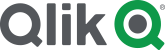#Community

Highlighted
Contributor

## Setting Multiple Variable Values at the one time

Hello there,

Using the Variable Input extension, I note it is possible to dynamically change the value of a single variable.

e.g. Variable1 is assigned a value based on a selection made by the user such as in a drop down box.

How do I set multiple variables at the one time:

 Chart Variable1 Variable2 A Total1 Line1 B Total2 Line2 C Total2 Line3

For example, if the user selects Chart A, then Variable 1 is set to 'Total1' and Variable2 is set to 'Line1'

Else I have expressions loaded in that are relying on the variable value

e.g.   Sum ( {< '\$(Variable1)' = {1}>} Sales)

Labels (2)

• ### Variables

1 Solution

Accepted Solutions
Contributor

## Re: Setting Multiple Variable Values at the one time

Hi Jordi,

Yes I couldn't quite work out how to use the Variable Input Extension twice.

In the end I did find a solution by creating an variable island in the data model  - loaded a table much like the one in my question.

And then referenced the variables via Set Analysis e.g.

Sum( {<\$(=FlagSelector) = {1}>}  \$(=TotalSelector) )

works a treat.

3 RepliesPartner

## Re: Setting Multiple Variable Values at the one time

Hi,

Have you already set one variable? Then you can do exactly the same for nr 2. But if the Variable Input Extension can only hold one variable, then you need that one twice.

Jordy

Climber

Work smarter, not harder
Contributor

## Re: Setting Multiple Variable Values at the one time

Hi Jordi,

Yes I couldn't quite work out how to use the Variable Input Extension twice.

In the end I did find a solution by creating an variable island in the data model  - loaded a table much like the one in my question.

And then referenced the variables via Set Analysis e.g.

Sum( {<\$(=FlagSelector) = {1}>}  \$(=TotalSelector) )

works a treat.Partner

## Re: Setting Multiple Variable Values at the one time

Hi,

Sounds good! Great that it worked. Please close the post.

Jordy

Climber

Work smarter, not harder